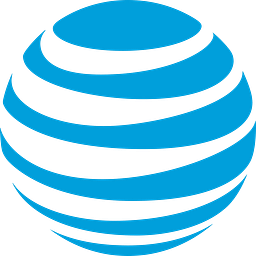# The Best Yielding Dow Jones Dividend Stocks In Review

+16.46%
Upside
16.57
Market
19.30
TrefisT
AT&T

This article was submitted by Dividend Yield using our Trefis Contributors tool

Best Yielding Dividend Stocks From Dow Jones Researched By Dividend Yields – Stock, Capital, Investment. The Dow Jones is a major index used by many investors. It is more important to know which companies pay the best dividends and how they are financed in order to pay these dividends. I screened stocks from the Dow Jones Industrial Average 30 Index by the best dividend yields and analyzed the financial situation. Finally, you find a little market valuation at the end of the company’s introduction.

Here are the best yielding Dow Jones 30 stocks:

Relevant Articles

1. AT&T (T) has a market capitalization of \$183.02 billion. The company employs 256,420 people, generates revenues of \$126,723.00 million and has a net income of \$4,184.00 million. The firm’s earnings before interest, taxes, depreciation and amortization (EBITDA) amounts to \$27,595.00 million. Because of these figures, the EBITDA margin is 21.78 percent (operating margin 7.27 percent and the net profit margin finally 3.30 percent).

The total debt representing 23.95 percent of the company’s assets and the total debt in relation to the equity amounts to 61.36 percent. Due to the financial situation, a return on equity of 3.63 percent was realized. Twelve trailing months earnings per share reached a value of \$0.66. Last fiscal year, the company paid \$1.73 in form of dividends to shareholders.

Here are the price ratios of the company: The P/E ratio is 46.71, P/S ratio 1.43 and P/B ratio 1.72. Dividend Yield: 5.75 percent. The beta ratio is 0.59.

2. Verizon Communications (VZ) has a market capitalization of \$109.65 billion. The company employs 193,900 people, generates revenues of \$110,875.00 million and has a net income of \$10,198.00 million. The firm’s earnings before interest, taxes, depreciation and amortization (EBITDA) amounts to \$29,376.00 million. Because of these figures, the EBITDA margin is 26.49 percent (operating margin 11.62 percent and the net profit margin finally 9.20 percent).

The total debt representing 23.93 percent of the company’s assets and the total debt in relation to the equity amounts to 153.33 percent. Due to the financial situation, a return on equity of 6.45 percent was realized. Twelve trailing months earnings per share reached a value of \$0.85. Last fiscal year, the company paid \$1.98 in form of dividends to shareholders.

Here are the price ratios of the company: The P/E ratio is 45.66, P/S ratio 0.98 and P/B ratio 3.03. Dividend Yield: 5.25 percent. The beta ratio is 0.55.

3. Merck & Co. (MRK) has a market capitalization of \$115.46 billion. The company employs 86,000 people, generates revenues of \$48,047.00 million and has a net income of \$6,392.00 million. The firm’s earnings before interest, taxes, depreciation and amortization (EBITDA) amounts to \$15,478.00 million. Because of these figures, the EBITDA margin is 32.21 percent (operating margin 15.26 percent and the net profit margin finally 13.30 percent).

The total debt representing 16.66 percent of the company’s assets and the total debt in relation to the equity amounts to 32.13 percent. Due to the financial situation, a return on equity of 11.49 percent was realized. Twelve trailing months earnings per share reached a value of \$2.03. Last fiscal year, the company paid \$1.52 in form of dividends to shareholders.

Here are the price ratios of the company: The P/E ratio is 18.71, P/S ratio 2.39 and P/B ratio 2.11. Dividend Yield: 4.40 percent. The beta ratio is 0.66.

4. Pfizer (PFE) has a market capitalization of \$161.40 billion. The company employs 103,700 people, generates revenues of \$67,425.00 million and has a net income of \$8,739.00 million. The firm’s earnings before interest, taxes, depreciation and amortization (EBITDA) amounts to \$23,011.00 million. Because of these figures, the EBITDA margin is 34.13 percent (operating margin 18.93 percent and the net profit margin finally 12.96 percent).

The total debt representing 20.72 percent of the company’s assets and the total debt in relation to the equity amounts to 47.39 percent. Due to the financial situation, a return on equity of 10.24 percent was realized. Twelve trailing months earnings per share reached a value of \$1.10. Last fiscal year, the company paid \$0.80 in form of dividends to shareholders.

Here are the price ratios of the company: The P/E ratio is 19.40, P/S ratio 2.40 and P/B ratio 1.98. Dividend Yield: 4.17 percent. The beta ratio is 0.71.

The best yielding stocks are still AT&T and Verizon. Both have a yield above five percent and pay stable dividends. The following stocks are still Merck and Pfizer. They belong to the major drug manufacturing industry – An investment field that suffers a little under patented losses.

Take a closer look at the full yield table of the Dow Jones. In average, dividend stocks from the Dow Jones have a yield of 2.84 percent. All 30 Dow Jones stocks pay dividends.

Selected Lists: